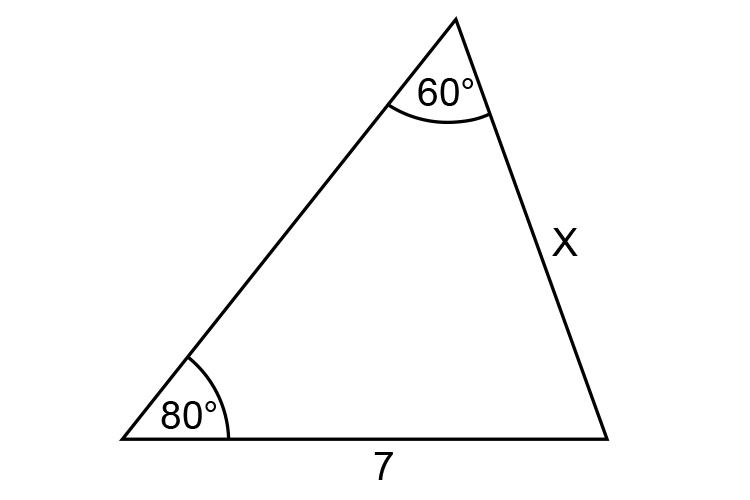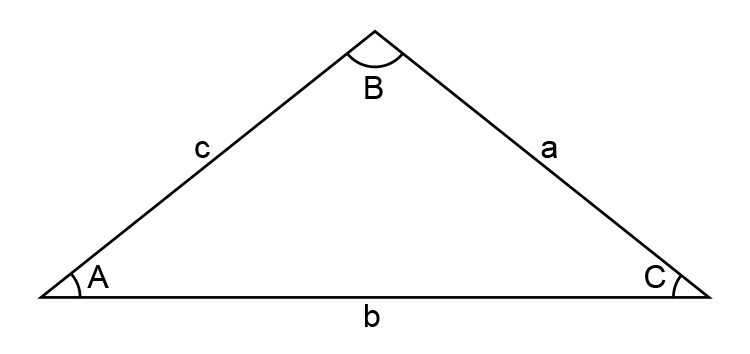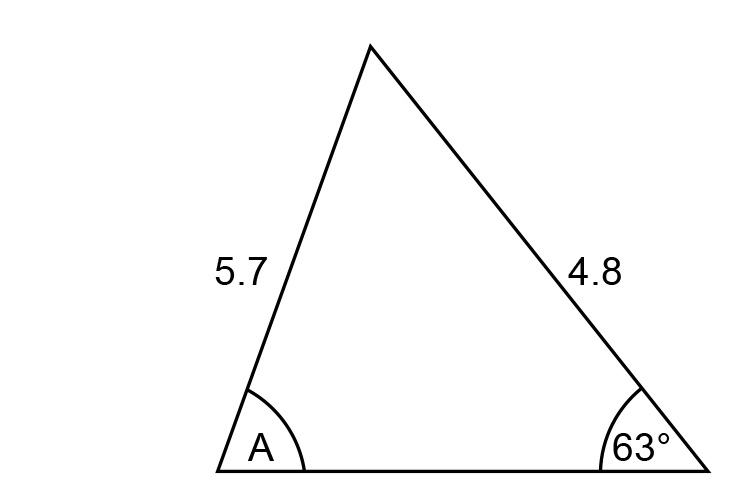# Examples using sine rule

You can use the sine rule (If you can remember it – but why try) here it is anyway:

Example 1

Find the length of x in the following diagram?Solution:

The sine rule: a/sinA=b/sinB=c/sinC

In a triangle that looks like:Therefore use:

 a/sinA = b/sinB a/sin80 = 7/sin60 a = (7timessin80)/sin60 a = 6.893/0.866 a = 7.959

Answer: x=7.959

Example 2

Find angle ASolution:

The sine rule: a/sinA=b/sinB=c/sinC

In a triangle that looks like:Therefore Use:

 a/sinA = c/sinC 4.8/sinA = 5.7/sin63 (4.8timessin63)/5.7 = sinA

Or

 sinA = (4.8timessin63)/5.7 sinA = 4.276/5.7 sinA = 0.75

Answer: A=48.61^@